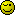# Preg match question - only one result is succesful

I am using the following code to look for semi-colons and commas in a \$subject. While each works individually, if I make a string (like “,;”)that combines semi-colon AND a comma, only the comma function works. (It reads “comma found”.)

``````
if (preg_match('/^;\$/', \$subject))
{
echo "semi colon found";
}

if (preg_match('/^,\$/', \$subject))
{
echo "comma found";
}

``````

Any ideas on identifying the problem here?

``````\$subject = ",;";

if (preg_match('/;/', \$subject))
{
echo "semi colon found";
}

if (preg_match('/,/', \$subject))
{
echo "comma found";
}
``````

semi colon found comma found

hope it helpsThat did the trick - thanks!

You (s|c)ould implement a character class instead.

``````
<?php
if(preg_match('~[,;]~', 'subject'))
{
echo 'comma or semi-colon found';
}

``````

You could also use the strpos function.

``````\$found = strpos(\$subject, ",") !== false || strpos(\$subject, ";") !== false;
``````